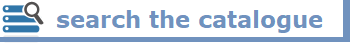# ✧  SciGator  ✧

### Explore University of Pavia libraries...⬉ home Browse subclasses ↓                           ...then launch a search by the buttons beside   [help] it / en
 ↑ `519.4` applied numerical analysis, finite elements, MATLAB→ `4 510.285 518 `↑ `004` hardware, networks, computer science generally→ `519.4  621.39  ≈ :P.0` ↑ `510.285` computers in mathematics→ `005.72  519.4` ↑ `518` numerical analysis→ `519.4` ↑ `624.17` structural systems, building science→ `519.4  620.1` ↑ `AN2` numerical analysis≈ `519.4` ↑ `AN3` approximation methods≈ `519.4` ↑ `AN4` numerical methods for partial derivative equations≈ `519.4` ↑ `AN5` numerical methods for ordinary differential equations≈ `519.4` ↑ `AN6` congresses (numerical analysis)≈ `519.4` ↑ `CS1` finite difference≈ `519.4` ↑ `CS2` padding≈ `519.4` ↑ `CS3` finite element≈ `519.4` ↑ `CS4` matrix methods≈ `519.4` ↑ `CS5` numerical methods≈ `519.4` ↑ `CS6` mathematical programming≈ `519.4` ↑ `CS7` conference (Calculation of structures)≈ `519.4` ↑ `D1D` Mathematics and mathematics manuals≈ `519.4` ↑ `D3A` Design and calculation of structures by electronic computer≈ `519.4` ↑ `ET7` numerical methods for electrical engineering≈ `519.4`  `621.3` ↑ `R4A` Numerical methods≈ `624.17`  `519.4` ↑ `R4B` Finite difference≈ `624.17`  `519.4` ↑ `R4C` Finite element≈ `624.17`  `519.4` ↑ `R4D` Mathematical Programming≈ `624.17`  `519.4` ↑ `R4E` Matrix methods≈ `624.17`  `519.4`